#Function Repository Resource:

# When

An operator form of Which

Contributed by: Taliesin Beynon
 ResourceFunction["When"][test1,value1,test2,value2,…][e] evaluates each of the testi[e] in turn, returning the value of the valuei corresponding to the first test that yields True; otherwise, it yields e unchanged.

## Details and Options

When must have at least one test and value.
ResourceFunction["When"] has attribute HoldRest.

## Examples

### Basic Examples

When evaluates the corresponding value only when the specified condition is met:

 In:=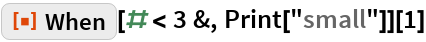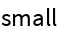In:=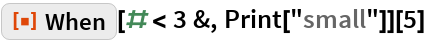Out=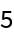### Scope

Use a single test with When:

 In:=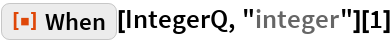Out=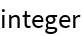In:=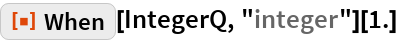Out=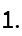Try several tests in turn:

 In:=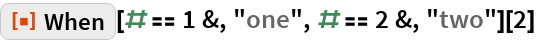Out=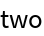In:=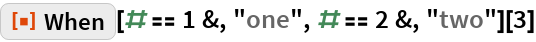Out=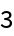### Properties and Relations

Which takes a test expression whereas When takes a test function:

 In:=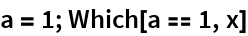Out=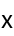In:=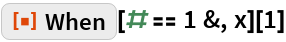Out=You can think of When as an operator form of Which:

 In:=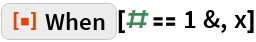Out=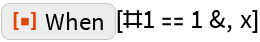In:=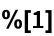Out=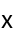## Requirements

Wolfram Language 11.3 (March 2018) or above